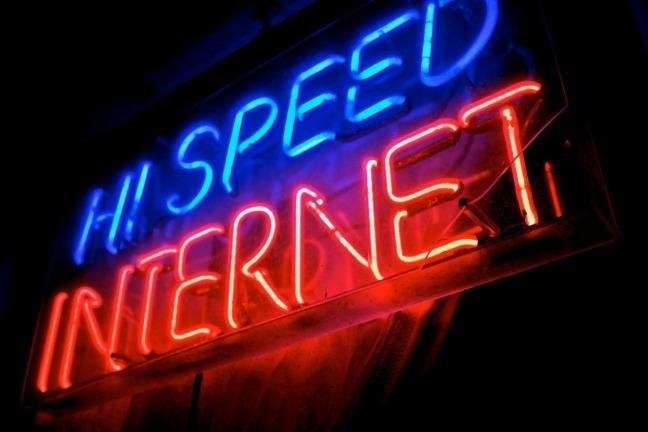1.6

## UNSW SydneyIt says hi speed, but how fast is it?

# How fast is your internet?

In this step you will learn

• differences between bits and bytes and what Mbps means

• how to handle different units of measurement.

## Internet speeds

If you remember dial-up, then you probably think all internet is fast. If you are from South Korea, you may be alarmed to discover that the rest of the world’s internet is very slow. These days with people wanting to download or stream high definition TV shows and movies, it’s useful to be able to apply some simple proportionalities.

So let’s move away from subjective statements about internet speed, and review basic facts we can all agree on.

## Megabytes and Megabits

In the world of computer science, numbers are not represented in base ten, but rather as binary digits: a sequence of zeros and ones. A single binary digit is called a bit. Eight bits is called a byte:

The term Mega means $\normalsize{ 10^6 }$ and can be used as a prefix to bytes or bits. So

while

These words are usually abbreviated as follows

Name Abbreviation
bit b
Byte B
Megabit Mb
Megabyte MB

The standard measurement for data transfer speed is in terms of Megabits per second (Mbps). So when someone says they can download at “$\normalsize{20}$ megs per second”, they mean Megabits; not Megabytes. Although sometimes even tech people get confused.

Visit the website http://www.speedtest.net and measure your internet speed. Notice that the measurements are in terms of Mbps. You can post a link to your results, or just tell us about it in the comments section. Please mention which country you are in. For example, here at UNSW Australia our download speed is $\normalsize{946.50}$ Mbps. That’s pretty fast, but it won’t be the fastest.

Suppose I want to watch a movie. The Social Network is available in High Definition (HD) or Standard Definition (SD). Since our internet speeds are all measured in terms of Megabits per second, let’s measure movie size in terms of Megabits so everything is consistent.

Movie Size Gigabytes Megabytes Megabits
HD 3.6 3600 28800
SD 0.7 700 5600

The basic relation is a direct proportionality:

When multiplying internet speed by download time, make sure your time and speed measurements are consistent. For example, multiply $3.36$ Mega bytes per second by a time measurement which is also in seconds (not minutes or hours).

Q1 (E): How long would it take to download The Social Network in HD using the average Australian internet speed $3.36 \text{ Mbps}$? What about in SD?

Q2 (E): If an SD movie is about 2 hours long, then what speed connection do you need to watch it without buffering?

Q3 (E): How long would it take you to download The Social Network in HD at your internet speed? Can you stream it?

If your speed is $\normalsize{12} \text{ Mbps}$ then that establishes a proportionality between download size and download time.

• If your Download Time is $\normalsize{1}$ minute, you can download $\normalsize{12 \times 60=720}$ Megabits, which is also $\normalsize{720/8=90}$ Megabytes.
• If your Download Time is $\normalsize{2}$ minutes, you can download $\normalsize{12\times120=1440}$ Megabits, which is $\normalsize{180}$ Megabytes.
• If your Download Time is $\normalsize{10}$ minutes, you can download $\normalsize{10}$ times as much as in $\normalsize{1}$ minute, namely $\normalsize{7200}$ Megabits.

The beauty of direct proportionality is that if you multiply the input by $\normalsize{10}$, then you multiply the output by $\normalsize{10}$, independent of the actual constant of proportionality.

A1. We need to solve the equation

$\normalsize{ 28800 = 3.36 \times \text{download time (in seconds)} }$.

Since ${\normalsize \frac{28800}{3.36} \approx 8571 }$ then download time is 8571 seconds, which is about the same as 2 hours and 23 minutes. For SD it only takes 28 minutes. This means that the average Australian cannot stream The Social Network in HD because the time it takes to watch the movie is less than the time it takes to download the movie. But SD is okay.

A2. We need to download the whole SD movie in under 2 hours. Since our speed is a rate per second, let’s first convert hours into seconds. $\normalsize 2$ hours is ${\normalsize 2 \times 60 \times 60 = 7200}$ seconds. So we need to solve the equation

$\normalsize{ 5600 = \text{download speed (in Mbps)} \times 7200 }$.

Since ${ \normalsize \frac{5600}{7200} \approx 0.78}$ then streaming in SD only requires a low internet connection speed of $0.78$ Mbps.

A3. See discussion.